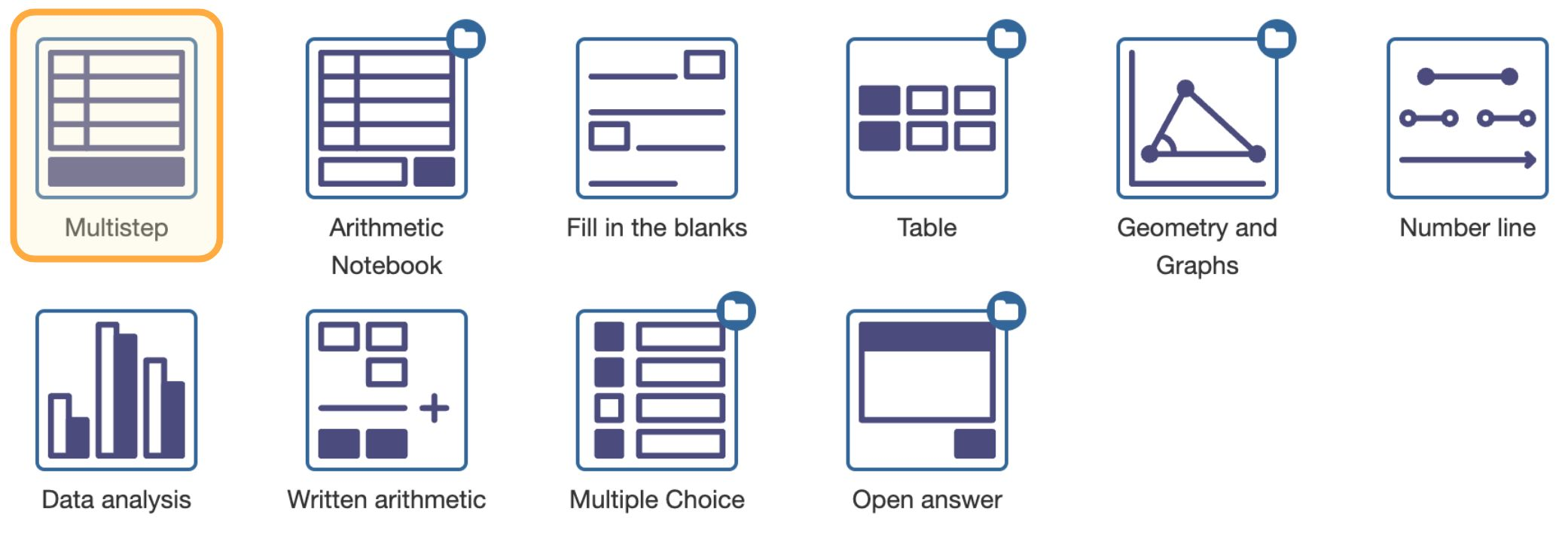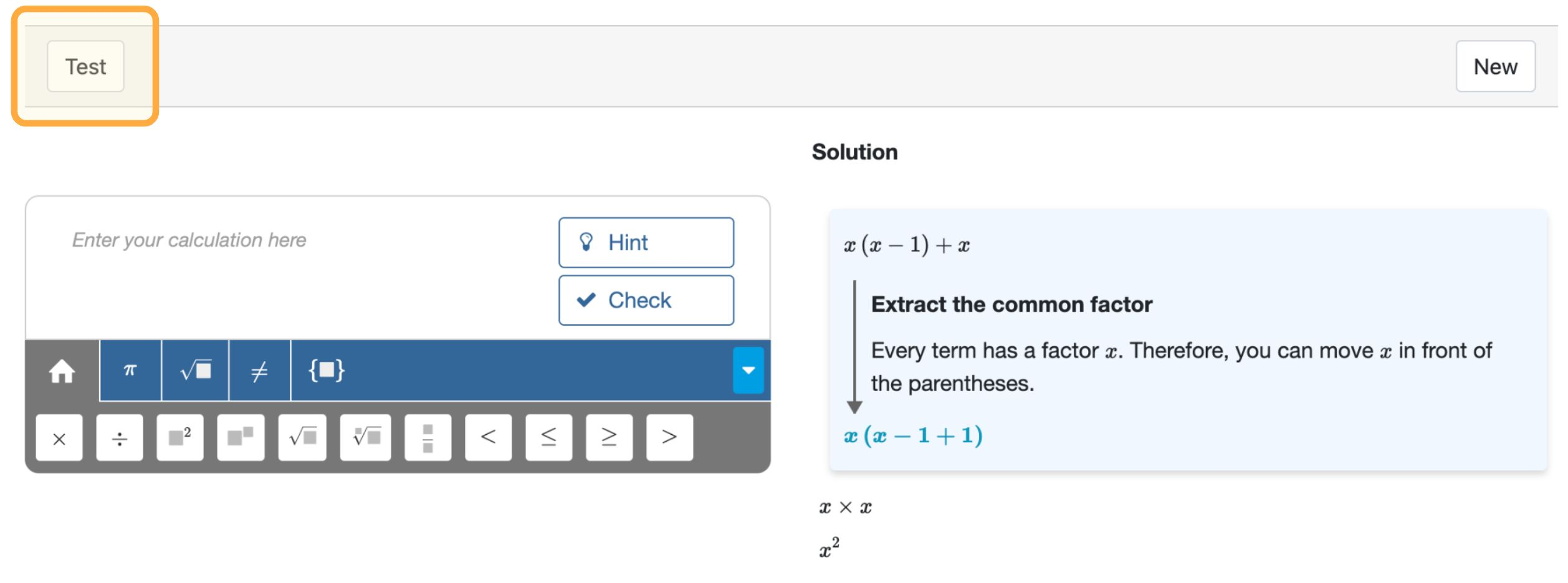## Define the problem, not the answer

The core feature of Multistep is its ability to evaluate substeps and give automated support. This is possible only if Multistep knows what problem students must solve. Therefore, you don't prescribe answers, but the tasks students should perform.

Example
• a task could be to simplify $\frac{2y^2\sqrt{5}}{xy}$. An answer is $2yx\sqrt{x}$.
• a task could be to solve $3(x-1)=2x+4$. An answer is $x=7$.

Algebrakit derives hints, error feedback, worked solutions, and answers from the task, so there is no need for you to input those. You can add custom hints or error feedback, as we will see later.

Multistep supports several tasks organized into the following categories:

• Simplifying expressions
• Performing numerical calculations, such as calculating percentages or converting units.
• Rewriting expressions, such as expanding, factoring, or rewriting expressions into different forms.
• Solving equations or inequalities
• Calculus, such as differentiation, integration, or calculating limits.

You can build more complex tasks from these. For example:

• Finding the equation of a line through two points, which requires:
1. Calculating the slope (Simplify).
2. Substitute coordinates to get the $y$-intercept (Solve).
3. Writing the equation (Simplify).
• Finding the coordinates of the maximum of a function, which requires:
1. Finding the derivative of the function (Differentiate)
2. Setting the derivative to zero and solving the equation (Solve)
3. Writing the coordinates of the maximum (Simplify)

How you create such composed tasks will be discussed in chapter Multistep (advanced)

Now let's create a new Multistep question. You can use the Testbench if you have no access to an Algebrakit-enabled content management system.Figure: Selecting the Multistep question type.

Click the icon for the Multistep question type to see the input form for Multistep. Click on the bar below 'Task' to expand it.Figure: The input form for Multistep

The default Task is to simplify an expression. In the input field, type $x(x-1)+x$.Figure: Configuring a task

Now press the Test button at the bottom of the page to see a preview of our question.Figure: Previewing our exercise.

The preview shows an interactive question on the left. You can answer the question step by step and request hints like a student would. The worked solution on the right shows how Algebrakit solves the problem. Click to expand the steps.

Note

See the table below if you are looking for information on the other input elements:

Simplifying is required with all tasks. Students must evaluate calculations and simplify expressions as much as possible.

• $1+1$ must be evaluated to $2$
• $x+y+x$ must be simplified to $2x+y$
• $x(x+1)-x$ must be simplified to $x^2$

Multistep will only rewrite an expression if that simplifies the result. For example, Multistep will not rewrite the following expressions:

• $x(x+1)$. An equivalent form is $x^2+x$.
Use the Expand task if you want the student to expand the expression.
• $\frac{1}{x}+\frac{2}{3}$. An equivalent form is $\frac{3+2x}{3x}$.
Use the Combine Fractions task if you want the student to combine the fractions.

Multistep will accept such an equivalent form as a final answer.

Simplifying does not include solving equations, so Multistep will not require students to rewrite $2x=4$ into $x=\frac12$. Use the Solve task if students should solve for $x$.

### Solving equations

In the category Solving Equations, you can find tasks for finding solutions to equations, inequalities, or systems of two equations.Figure: Task: Solving Equations

You can use the "Solving Equations: Single Variable" task to solve a wide variety of relations. The table shows some examples:

Relation Result Explanation
$6(p-1)=4p+10$ $p=8$ A linear equation
$a\cdot x=b$ $x=\frac{b}{a}$ Algebraic solutions are allowed
$2(1-x)\leq4$ $x\geq -1$ Inequalities are supported
$\frac{1}{\sqrt{1-x}}>1$ $0 Algebrakit keeps track of the domain
$2\sin \left(2x-\frac{1}{4}\pi \right)=\sqrt{2}$, $0 $x=\frac{\pi}{4}, x=\frac{\pi}{2}$ Trigonometry
$\begin{cases}2x-1=y\\x+y=1\end{cases}$ $\begin{cases}x=\frac{2}{3}\\y=\frac{1}{3}\end{cases}$ System of two equations

As always, Multistep offers support through hints and substep evaluations. You can try them here:

Note

The following type of equations are not yet supported

• Systems of more than two equations
• Systems of inequalities
• Equations with solutions in the complex plane

We invite you to check out the other tasks in the Multistep editor. Most of them will be self-explanatory. The table below shows some examples.

Expand $x(x-1)$ $x^2-x$
Factor $x^2-x$ $x(x-1)$
Combine Fractions $\frac{1}{x}+\frac{2}{3}$ $\frac{3+2x}{3x}$
Complete the Square $x^2+6x+7$ $\left(x+3\right)^2-2$
Differentiate $\sin\left(2x^2\right)$ $4x\cos\left(2x^2\right)$
Integrate $4x\cos\left(2x^2\right)$ $\sin\left(2x^2\right) + c$

## Custom feedback

Although Multistep can show error feedback for some common mistakes, you sometimes want to add custom error feedback. You can achieve this by adding an incorrect task.Figure: Adding a task. Hover your mouse over the task to see these task buttons

Use the dropdown in the new task to indicate that this activity is incorrect. You can add custom error feedback as well.Figure: Specifying custom feedback in an "incorrect" task

Algebrakit will show the feedback when a student inputs an expression that matches this task.## Custom hints

You can also add your custom hints to tasks. Algebrakit will show these custom hints before any generated hints.Figure: Adding a custom hint to a taskFigure: Two hints in the Multistep question. Algebrakit shows the custom hint before the generated hint.